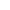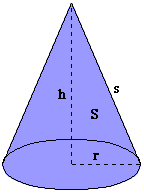Volume Of Cones And Spheres Cone Volume A cone is a geometric figure generated by a group of all lines through a given point (called the vertex) and passing through a curve in a plane (this is called the directrix, which we know as the circular base of the cone).  A cone also includes the solid enclosed by a cone and the plane of the directrix. The region of the plane enclosed by the directrix is called a base of the cone.  The perpendicular distance from the vertex to the plane of the base (known as the axis) is the height of the cone. There are a few different types of cones, including some for which the base is not a circle, but a different base, almost like a toddler attempting to draw a circle might come up with.  However, our knowledge of geometry does not allow us to understand without some of the higher math the formula and surface area of such figures.  For this site, I will focus on the volumes of the cones that are and circular.  Of these, there are 2 types of such cones: oblique and right circular cones.  Right circular cones are those where the axis is perpendicular to the plane of the base and the base is circular.  Oblique circular cones are those cones where the base is circular, but the axis (height) is not perpendicular to the base.  These cones both share a simple formula for volume: Volume of a circular cone = (Pi · r^2 · h)(1/3) In this formula: - r =  radius of the circular base of the cone; - h = perpendicular height from the plane of the base to the vertex of the cone. Here's how it works: We know that the volume of a cone is (1/3 · Pi · r^2 · h).  But why does this formula work?  There's a reason and it can be proved, but not without using calculus!  However, there is a way to demonstrate it.  Visualize a cone in your mind, first.  For every cone, there is always a cylinder with equal height and base area.  Now, visualize the cylinder and your cone inside of it.  If you pour the cone's volume in water into the cylinder, your cylinder won't get filled up all the way.  Now do it again - and trust me on this- and your cylinder still isn't filled all the way up with water.  Do it a third time, however, and your cylinder will be filled all the way up with water!  So, if we're given that the volume of a cylinder is (Pi ·r^2 · h), then we should be able to find the volume of the cone in relation to a cylinder with equal base area and height.  Because the cone had to empty all of its volume three separate times into the cylinder, it must be true that the volume of the cone is 3 times that of the cone. Conversely, the volume of the cone is 1/3 of that of the corresponding cylinder with equal height and base area.  Every cone has a corresponding cylinder; therefore, the volume of the cone must be (1/3 · Pi · r^2 · h)! Here's an example:Given: height = 10 m; radius = 4 m Find the volume of the cone at left using 3.14 for Pi. V(cone) = (Pi · r^2 · h) (1/3) V(cone)  = [(3.14) · (4)^2 · (10)] (1/3) V(cone)  = [(3.14) · (16) · (10)] (1/3) V(cone)  = [50.24 · 10] (1/3) V(cone)  = [502.40] (1/3) V(cone)  = 165.79 m Sphere Volume A sphere is a figure defined by all points that are a given distance from the center, the point around which all the others are arranged.  They are often in everyday life, in shapes such as baseballs and basketballs.  There really isn't much to say about them except for the fact that they are the most common geometric shape (in my life)! Volume of a sphere = (4/3 · Pi) (r^3) In this formula: -r = radius of the sphere Here's how it works: We know that the volume of a sphere is (4/3 · Pi · r^3).  But why does this formula work?  First off, to actually prove it, you have to know calculus--so I guess that option is out!  However, it is possible for you to figure it out by demonstrating it and using a little math.  Start by visualizing a hemisphere (half of a sphere) in your mind.  Now visualize a cylinder with a height of twice the radius of the sphere, and a base equal in area to the cone's base.  Fill the hemisphere with water and then pour it into the cylinder.  You'll have to do this three times. (I know -- I've tried it!)  Now, we know that the volume of a hemisphere is 1/3 of the volume of a cylinder with the same base area and two times the height.  To work it out the rest of the way, we'll need to use substitution a few times, as well as the formula for volume of a cylinder.  The volume of a cylinder is (Pi · r^2 · h).  Because we are using the volume of the hemisphere as our base for the equation, we'll use "r" to represent the radius of the hemisphere.  Since the height of the cylinder was two times the radius of the hemisphere, the height can be represented as 2r.  Therefore, Volume(cylinder) = Pi · r^2 · h Volume(cylinder) = Pi · r^2 · (2r) Volume(cylinder) = Pi · (2r^3) Volume(cylinder) = 2r^3 · Pi Volume(cylinder) = 2 · Pi · r^3 Now, we have the volume of the cylinder expressed in terms of the hemisphere's radius. We can use this to solve for the volume of the hemisphere. Since the volume of the hemisphere is 1/3 of the volume of the cylinder, we can plug that in and solve for the volume of the hemisphere.  So, Volume(hemisphere) = (1/3) Volume(cylinder) Volume(hemisphere) = (1/3) (2 · Pi · r^3) Volume(hemisphere) = (2/3 · Pi · r^3) Finally, we have the volume of the hemisphere, but that's only half of the sphere (hence, "hemi"), so we have to multiply the hemisphere's volume by 2 to find the volume of the entire sphere.  So, you multiply 2/3 by 2/1 (which is equal to 2) to find the sphere's volume.  You find that the volume of the sphere is equal to 4/3 · Pi · r3!! Here's an example: Given:  r = 3 cm.Find:  The volume of the sphere, using 3.14 for Pi. Volume(sphere) = (4/3 · Pi) (r^3) Volume(sphere) = (4/3 · Pi) ((3)^3) Volume(sphere) = (4/3 · Pi) (27) Volume(sphere) = (36 · Pi) Volume(sphere) = 113.04 cm Home